# Surface Area of 3D Shapes

GCSELevel 4-5Level 6-7Edexcel iGCSE

## Surface Area of 3D Shapes

The surface area of a 3D shape is a measure of the total area of all the surfaces of that shape.

When calculating the surface area of 3D shapes, they can fall into one of the following categories:

• All the surfaces are flat – e.g. cube, cuboid, prism
• Some of the surfaces are curved – cylinder, sphere, cone

## Surface Area of Cubes and Cuboids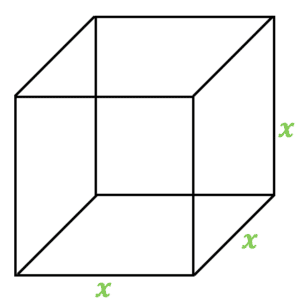A cube is made of $\textcolor{#00d865}{6}$ identical square faces, i.e. all the dimensions of the cube are the same.

Area of one face : $\textcolor{#00d865}{x} \times \textcolor{#00d865}{x} = \textcolor{#00d865}{x^2}$

Surface area of a cube :

$\boxed{6\textcolor{#00d865}{x^2}}$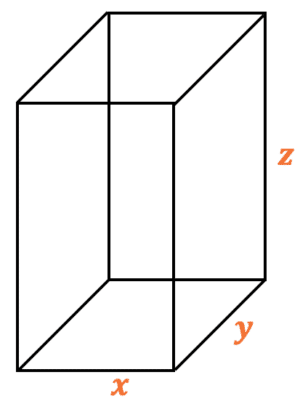A cuboid is made up of $\textcolor{#f95d27}{6}$ rectangular faces, where the opposite faces are identical, i.e. we have $\textcolor{#f95d27}{3}$ pairs of differently sized rectangles.

Area of the rectangles :

1. The first rectangle has sides $\textcolor{#f95d27}{x}$ and $\textcolor{#f95d27}{y} \rightarrow \textcolor{#f95d27}{xy}$
2. The second rectangle has sides $\textcolor{#f95d27}{x}$ and $\textcolor{#f95d27}{z} \rightarrow \textcolor{#f95d27}{xz}$
3. The third rectangle has sides $\textcolor{#f95d27}{y}$ and $\textcolor{#f95d27}{z} \rightarrow \textcolor{#f95d27}{yz}$

Since there are $2$ of each of these rectangles, the total surface area of cuboid is:

$\boxed{2(\textcolor{#f95d27}{xy}+\textcolor{#f95d27}{xz}+\textcolor{#f95d27}{yz})}$

Level 4-5GCSEEdexcel iGCSE

## Surface Area of a Cylinder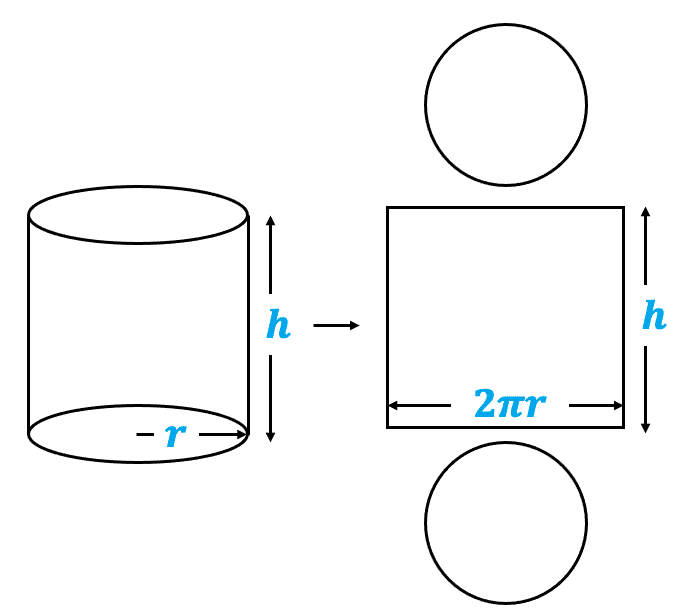A cylinder is made up of $2$ circles and one rectangle, shown in the diagram to the left.

Area of a circle : $\textcolor{#10a6f3}{\pi r^2}$

Circumference of a circle : $\textcolor{#10a6f3}{2 \pi r}$

$\textcolor{#10a6f3}{r} \rightarrow$ radius of the circle

Area of rectangle : circumference $\times$ height of cylinder $= \textcolor{#10a6f3}{2 \pi rh}$

Surface area of a cylinder :

$\boxed{\textcolor{#10a6f3}{2 \pi rh} + \textcolor{#10a6f3}{2 \pi r^2}}$

Level 4-5GCSEEdexcel iGCSE

## Surface Area of a Prism

A prism is a 3D shape that has identical faces at both ends, i.e. the shape has a constant cross-section (shaded faces in the diagram below) in one particular direction. Take a look at the examples below: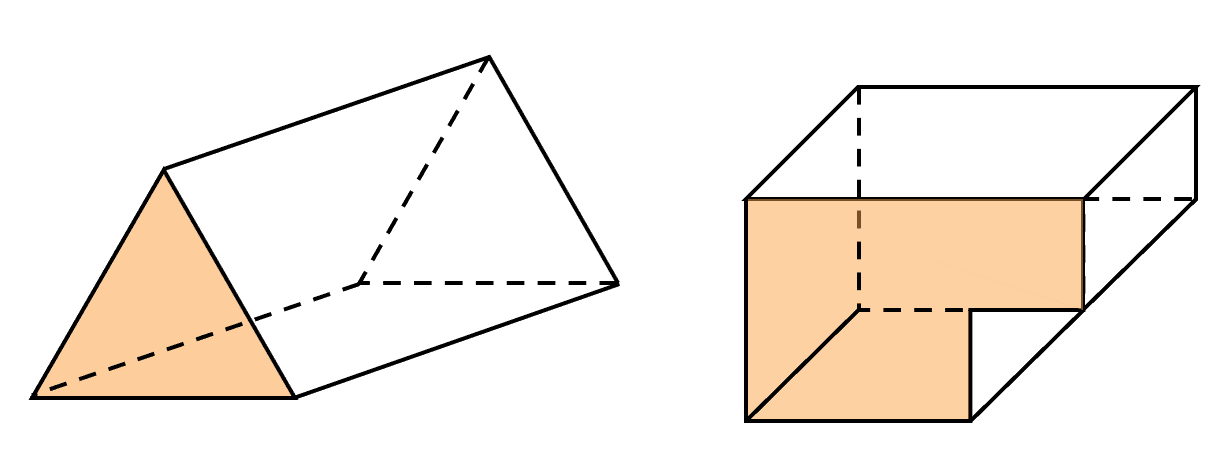To calculate the area of any prism you need to work out the area of the cross-section and any of the remaining faces.

Reminder: Area of a triangle : $\dfrac{1}{2}bh \,$ Where $b \rightarrow$ base of the triangle and $h \rightarrow$ height of the triangle

Surface area of an (isosceles) triangular prism :

$\boxed{\textcolor{#ffad05}{bh} + \textcolor{#ffad05}{2ls} + \textcolor{#ffad05}{bl}}$

$\textcolor{#ffad05}{l} \rightarrow$ length of shape

$\textcolor{#ffad05}{s} \rightarrow$ slanted length of triangle

Level 4-5GCSEEdexcel iGCSE

## Surface Area of a Cone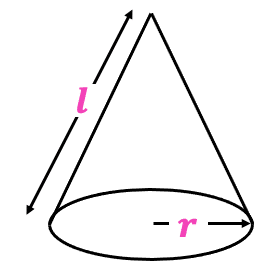A cone is made up of a circular base and a curved face joining to the circle.

Area of a circle : $\textcolor{#f21cc2}{\pi r^2}$

Area of the curved face : $\textcolor{#f21cc2}{\pi rl}$

$\textcolor{#f21cc2}{r} \rightarrow$ radius of the circle

$\textcolor{#f21cc2}{l} \rightarrow$ slanted height of the cone

Surface area of a cone :

$\boxed{\textcolor{#f21cc2}{\pi r^2} + \textcolor{#f21cc2}{\pi rl}}$

Level 6-7GCSEEdexcel iGCSE

## Surface Area of a Sphere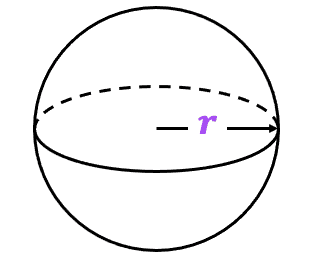Surface area of a sphere :

$\boxed{\textcolor{#aa57ff}{4\pi r^2}}$

$\textcolor{#aa57ff}{r} \rightarrow$ radius of the circle

Level 6-7GCSEEdexcel iGCSE

## Example 1: Cube and Cuboid

The surface area of cuboid $A$ is $108 \text{ cm}^2$

The surface area of cuboid $A$ is twice the size of the surface area of cube $B$

What is the width of cube $B$?

[3 marks]

Surface area of cube $B = 108 \div 2 = 54 \text{ cm}^2$

Let’s say cube $B$ has dimensions $x \text{ cm}$

Then we have,

$6x^2=54$

$x^2=9$

$x=3$

The width of cube $B$ is $3 \text{ cm}$

Level 4-5GCSEEdexcel iGCSE

## Example 2: Cylinders

Work out the surface area of a cylinder with radius $3 \text{ cm}$ and height $10 \text{ cm}$

Give your answer in terms of $\pi$

[2 marks]

Surface area of a cylinder: $2\pi r^2 + 2\pi rh$

Substituting $r=3$ and $h=10$,

Surface area of a cylinder: $2\pi \times 3^2 + 2\pi \times 3 \times 10 = 78\pi \text{ cm}^2$

Level 4-5GCSEEdexcel iGCSE

## Example 3: Cone

A cone with radius $4 \text{ cm}$, has a surface area of $40\pi \text{ cm}^2$

Work out the slanted height of the cone

[3 marks]

Surface area of a cone: $\pi r^2 + \pi rl$

Substituting $r=4$,

$\pi \times 4^2 + \pi \times 4l = 40\pi$

$16 \pi + 4l \pi = 40\pi$

$4l \pi = 24\pi$

$l = 6$

The slanted height of the cone is $6 \text{ cm}$.

Level 6-7GCSEEdexcel iGCSE

## Example 4: Hemisphere

Shown below is a hemisphere with radius $5 \text{ cm}$.

Calculate the surface area of the hemisphere.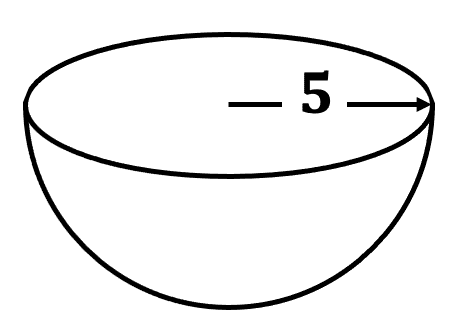Area of a circle with radius $5 \text{ cm}$ : $\pi \times 5^2 = 25 \pi \text{ cm}^2$

Area of a sphere with radius $5 \text{ cm}$ : $4 \times \pi \times 5^2 = 100 \pi \text{ cm}^2$

Area of a hemisphere with radius $5 \text{ cm}$ : $\dfrac{100 \pi}{2} + 25 \pi= 75 \pi \text{ cm}^2$

Level 6-7GCSEEdexcel iGCSE

## Example 5: Triangular Prism

Work out the surface area of the triangular prism below. Give your answer to two decimal places.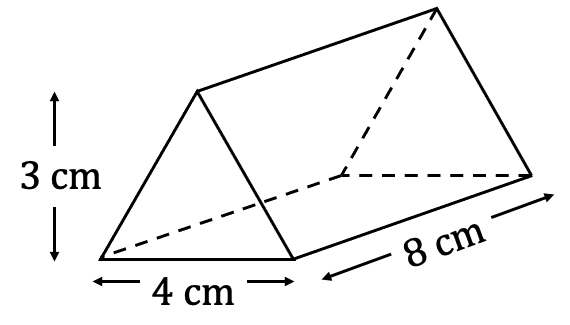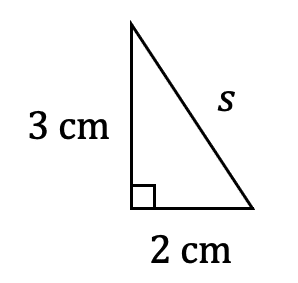To calculate the area of the two identical rectangles remaining, we first need to calculate the length of the slanted side of the triangle. To do this we use Pythagoras:

$s^2=2^2+3^2 = 13$

$s = \sqrt{13}$

Area of the side rectangles : $\sqrt{13} \times 8 = 8\sqrt{13} \text{ cm}^2$

Surface area of triangular prism : $8\sqrt{13} + 8\sqrt{13} + 32 + 6 + 6 = 44 + 16\sqrt{13}$ or $101.69 \text{ cm}^2$

Level 4-5GCSEEdexcel iGCSE

## Surface Area of 3D Shapes Example Questions

Radius of the cylinder : $6 \text{ cm}$

Surface area of a cylinder : $2 \pi rh + 2 \pi r^2$

Substituting $r=6$,

$2\pi \times 6^2 + 2\pi \times 6 \times h = 168\pi$

$72\pi + 12\pi h = 168\pi$

$12\pi h = 96\pi$

$h = 8$

The height of the cylinder is $8 \text{ cm}$

Surface area of a cuboid : $2(xy+xz+yz)$

Substituting $x=5$ , $z=6$ and $y=10$

$2(5\times 10+5\times 6+6\times 10) = 280 \text{ cm}^2$

Surface area of a sphere : $4\pi r^2$

$4\pi r^2=36\pi$

$r^2=9$

$r=3$

The sphere has a diameter of $6 \text{ cm}$

## You May Also Like...### MME Learning Portal

Online exams, practice questions and revision videos for every GCSE level 9-1 topic! No fees, no trial period, just totally free access to the UK’s best GCSE maths revision platform.

£0.00